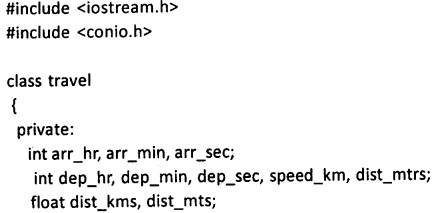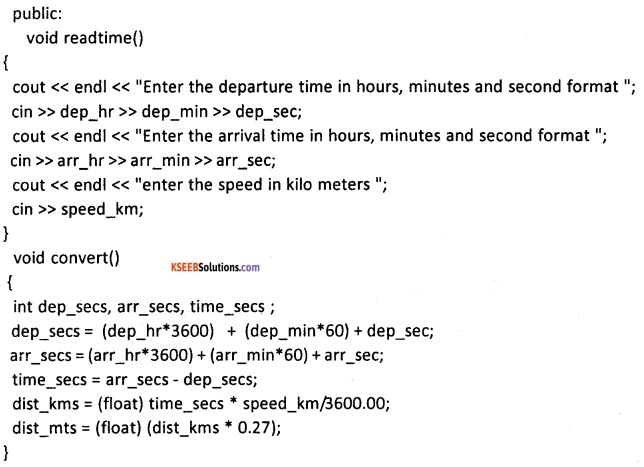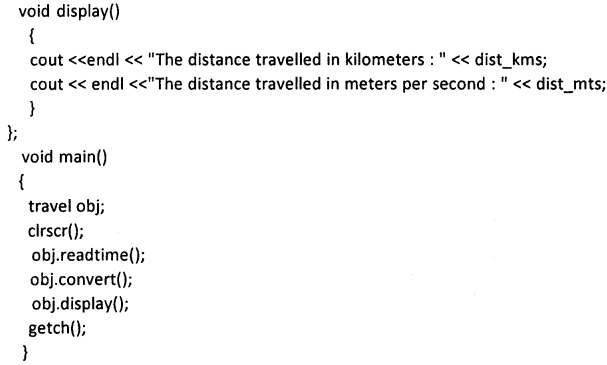# 2nd PUC Computer Science Question Bank Chapter 7 Classes and Objects

You can Download Chapter 7 Classes and Objects Questions and Answers, Notes, 2nd PUC Computer Science Question Bank with Answers Karnataka State Board Solutions help you to revise complete Syllabus and score more marks in your examinations.

## Karnataka 2nd PUC Computer Science Question Bank Chapter 7 Classes and Objects

### 2nd PUC Computer Science Classes and Objects One Mark Questions and Answers

Question 1.
What is a class?
Answer:
class is a structured data type in C++ which is a collection of variables and functions.

Question 2.
What is an object?
Answer:
The object is an instance of a class.

Question 3.
What are the two types of members referenced in a class?
Answer:
The two types of member referenced in a class are data members and member functions.Question 4.
What are data members?
Answer:
The variables are declared inside the class and are called data members.

Question 5.
What is a member function?
Answer:
The member functions are behaviour of a class and can access or manipulate data members without passing them as parameters.

Question 6.
Mention the access specifiers used with a class.
Answer:
The access specifiers used with a class are private, protected and public.

Question 7.
Is it possible to access data outside a class?
Answer:
Yes, the public members can be accessed by member functions of the class and non member function (outside the class) of the class.

Question 8.
Which type of data members are accessible outside a class?
Answer:
The public members can be accessed by member functions of the class and non member function (outside the class) of the class.Question 9.
Which access specifier is implicitly used in a class?
Answer:
The private access specifer is implicitly used in a class.

Question 10.
Define the term public access.
Answer:
The public members can be accessed by member functions of the class and non member function (outside the class) of the class.

Question 11.
Mention the operator used to access members of a class?
Answer:
The dot (.) is used to access members of a class.

Question 12.
What is the significance of scope resolution operator?
Answer:
The operator :: known as scope resolution operator helps in defining member function outside the class.

Question 13.
How are objects of a class declared? Give an example.
Answer:
Syntax: classname objectname1, objectname2, …. ,objectnamen ;
For example, Largest ob1,ob2; //object declarationQuestion 14.
What is meant by an array of objects?
Answer:
The array of class type is known as array of objects.

Question 15.
Write an example to show how objects can be used as function arguments?
Answer:
For example,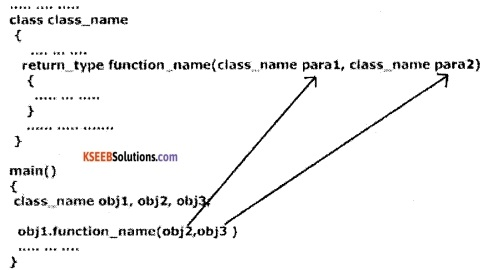### 2nd PUC Computer Science Classes and Objects Two Marks Questions and Answers

Question 1.
Write the differences between class definition and class declaration.
Answer:
A class definition is a process of naming a class and data variables and interface operations of the class. A class declaration specifies the representation of objects of the class and set of operations that can be applied to such objects.

Question 2.
Write the syntax and example for class definition.
Answer:
The syntax of a class definition: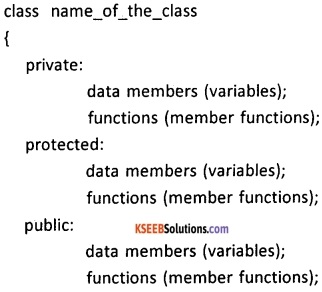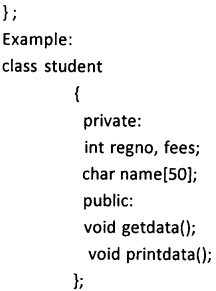Question 3.
Write the syntax and example for class declaration.
Answer:
Syntax: classname objectname1, objectname2, …. ,objectname………… n ;
For example, Largest ob1,ob2; //object declaration will create two objects ob1 and ob2 of largest class type.

Question 4.
What is the significance of using access specifiers? Mention different access specifiers.
Answer:
The data members and member functions can be accessed using access specifiers. They define the scope of members. The different access specifiers are private, protected and public.Question 5.
Discuss private access specifiers with an example.
Answer:
Private members:
The member data and members functions defined using access specifier private, can be accessed by member functions of that class only. Non-members of the class cannot access private members of the class. If no access specifier is mentioned for the members, then it is treated as private members.
For example;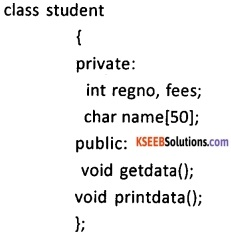The data members regno, fees and name can be accessed by only getdata() and printdata() member functions of the class student.

Question 6.
Write short notes on public access specifiers.
Answer:
The public members of a class can be accessed by member functions of that class and also non member functions (outside the class) of the class. The public member functions can access private, protected and public data members of the class.

Question 7.
Explain protected access.
Answer:
The protected members are similar to private members of a class in a single class. But, if a class is derived from an existing class, then protected members of the base class are accessible to the derived class.

Question 8.
How are class members referenced? Discuss with suitable example.
Answer:
The class members are referenced using dot (.) operator. The public data members of a class can be accessed using dot (.) operator using objects of that class.
For example, objectname . datamember;
The member function is invoked by a object name dot operator and member function name.
For example, obj1.getdata();

Question 9.
What is meant by referencing member functions inside class definition and outside class definition?
Answer:
In C++, the member functions can be coded in two ways :

1. Inside class definition
2. Outside class definition using scope resolution operator (::)

The code of the function is same in both the cases, but the function header is different as explained below:
1. Inside Class Definition:
When a member function is defined inside a class, we do not require to place membership label along with the function name. We use only small functions inside the class definition and such functions are known as inline functions.
In case of inline function, program execution is faster but memory penalty is there.

2. Outside Class Definition Using Scope Resolution Operator (::)
The member function declared in the class must be defined outside the class. Here the operator :: known as scope resolution operator helps in defining the member function outside the class.
In this case the function’s full name (qualified_name) is written as shown: Name_of_the_class :: function_name()
The syntax for a member function definition outside the class definition is:
retum type name_of_the_class::function_name (argument list)
{
body of function;
}Question 10.
Discuss how objects of a class are referenced with an example.
Answer:
The objects of a class can be referenced by passing objects as function arguments. The passing objects as function arguments can be pass by value or pass by reference.
Example;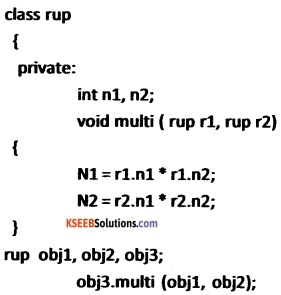In the above example, rup is the class, n1 and n2 are data members, multi() is member function with r1, r2 are the function argument of object type. The line obj3.multi (obj1, obj2); pass obj1 and obj2 which are the objects of class rup type. This type of function call is called call by value.

Question 11.
How can arrays be used as class members. Write an example.
Answer:
The arrays can be used as data members and objects as well. For example, class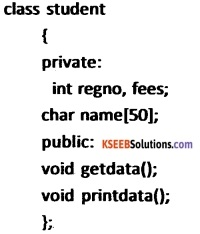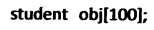In the above example, data member name is an character array and obj is an array of objects.

Question 12.
How are objects passed as arguments to a function? Give an example.
Answer:
The function arguments can be objects and passed in two ways namely pass by value and pass by reference. In pass by value, a copy of the object as actual argument is passed to the function and formal arguments of function which of class type receive the copy of the actual argument and process it.

Any changes made in the formal argument inside the function body will not reflect in actual arguments.
In pass by reference, the address of an object is passed as actual argument and formal argument holds the address of objects of actual arguments. Any changes made to formal argument in the function body will reflect in the actual argument since it holds the actual address of actual argument objects.
The pass by value example:
The objects of same class can be passed as arguments to a function.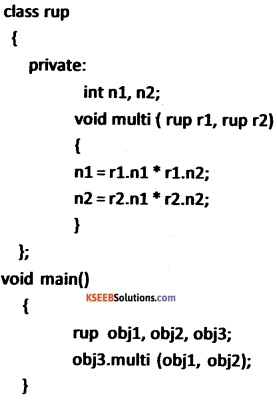The line obj3.multi (obj1, obj2); pass obj1 and obj2 which are the objects of class rup type.

### 2nd PUC Computer Science Classes and Objects Five Mark Questions and Answers

Question 1.
Explain class definition and class declaration with syntax and example.
Answer:
A class is a structured data type in C++ which is a collection of variables and functions. A class in C++ combines related data and functions together. It makes a data type which is used for creating objects of this type
The syntax of a class definition: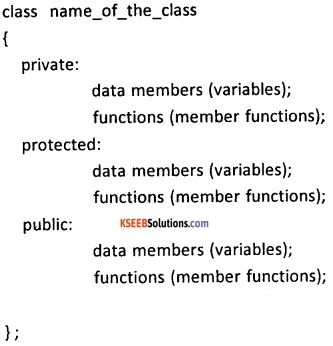Where

1. Class is a keyword in C++
2. name_of_the_class is the name of the class and can be treated as the user defined data type.
3. The pair of flower braces indicates the body of the class
4. The semicolon (;) after the right brace is must.
5. The variables are declared inside and are called data members
6. The member functions can access or manipulate data members without passing them as parameters.
7. The private,protected and public are access specifiers for the members of class.

Syntax of object declaration:
classname objectname1, objectname2, …..,
Example;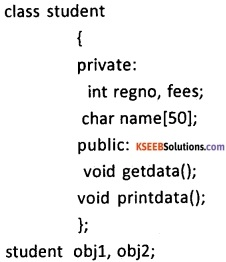In the above example, student is a class haveing regno, fees and name as data members. The getdata() and printdata() are the members functions of the class. The obj1 and obj2 are objects of class student.Question 2.
Describe access specifiers in a class.
Answer:
The data members and member functions can be accessed using access specifiers. They define the scope of members. The members of class are classified based on private/public/protected members.
1. Private members:
The member data and members functions defined using access specifier private, can be accessed by member functions of that class only. Non-members of . the class cannot access private members of the class. If no access specifier is mentioned for the members, then it is treated as private members.

2. Protected members:
The protected members are similar to private members of a class in a single class. But, if a class is derived from an existing class, then protected members of the base class are accessible to the derived class.

3. Public members:
The public members can be accessed by member functions of the class and non member function (outside the class) of the class. The public member functions can access private, protected and public data members of the class.

Question 3.
Explain member function

1. inside class definition
2. outside class definition

Answer:
Class member definition describes how certain class member functions are coded.
In C++, the member functions can be coded in two ways :

1. Inside class definition
2. Outside class definition using scope resolution operator (::)

The code of the function is same in both the cases, but the function header is different as explained below:
1. Inside Class Definition:
When a member function is defined inside a class, we do not require to place a membership label along with the function name. We use only small functions inside the class definition and such functions are known as inline functions.

2. Outside Class Definition Using Scope Resolution Operator (::):
The member function is declared inside the function and defined outside the class using the scope resolution operator.
The syntax for a member function definition outside the class definition is :
retum_type name_of_the_class::function_name (argument list)
{
body of function
}
Here the operator :: known as scope resolution operator helps in defining the member function outside the class.
Example;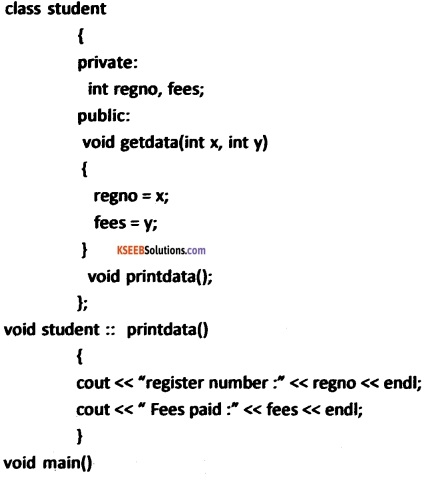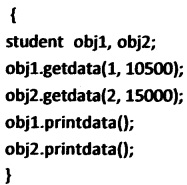Question 4.
What are the characteristics of member function outside a class?
Answer:
The member functions declared outside a class has the following characteristics:

1. Data type and number of argument in member function must be same as data types and number of data declared in class definition.
2. Type scope resolution operator (::) helps in defining the member function outside the class. It also identifies the function as a member of particular class.
3. The different classes can use same member function names. Membership label will resolve their scope limiting a particular class.
4. Member function can access private and protected data of a class, but not a non-member function.

Question 5.
Explain how objects of a class can be defined?
Answer:
The objects are declared after a class is defined. The object declaration create object of that class and memory is allocated for the created object. The object are created using the following syntax:
classname objectname1, objectname2,……………;
for example
student obj1, obj2;
the obj1 and obj2 are the objects of class type student.
The object names are used to access the data members through member functions by invoking member functions.
For example
obj1.getdata(5, 25);
here, getadata() is the member function of a class student and function call is give using object obj1 which assign the value 5 and 25 to the data members of the class. The memory is allocated separately for data members of each object.

Question 6.
Illustrate how an array of objects can be defined.
Answer:
The arrays can be used as data members and objects as well.
For example,
class student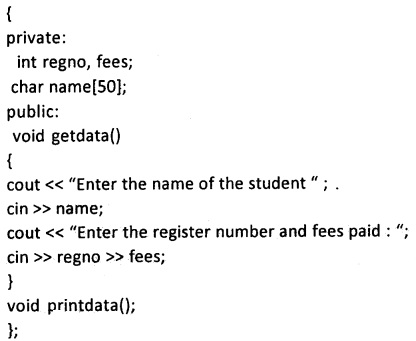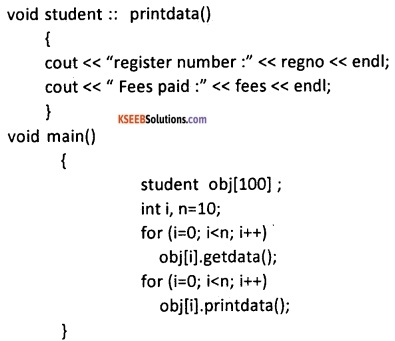in the above example, data members are name, regno, and fees are data members. The getdata() and printdata() are the members functions of the class student and obj is an array of objects of type class student. The for loop is used to pass value to data member for all the objects and another time for loop is used to print the data values of data members of the class.

Question 7.
Describe how objects can be used as function arguments.
Answer:
The objects of same class can be passed as arguments to a function. This is explained with an example,
class rup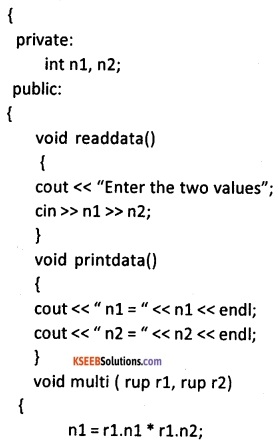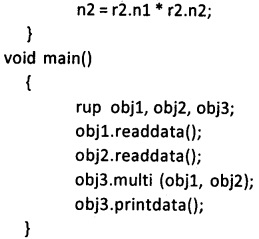in the above example, rup is the class, n1 and n2 are data members, readdata(), printdata() and multi() are member functions with r1, r2 are the function argument of object type.
The line obj3.multi (obj1, obj2); pass obj1 and obj2 which are the objects of class rup type. In the statement
n1 = r1.n1 * r1.n2;
n2 = r2.n1 * r2.n2;
r1.n1 is accessing data member n1 of object r1 and r1.n2 is accessing data member n2 of object r1 and their product is stored in data member n1 and same for n2 in the second line which is the member of obj3 object.
The obj3.printdata() gives out the result stored in the n1 and n2.Question 8.
Let product list be a linear array of size N where each element of the array contains following fields itemcode, price and quantity. Declare a class product list with the three data members and member functions to perform the following:
Answer:

1. Add value to the product list.
2. printing the total stock value, class product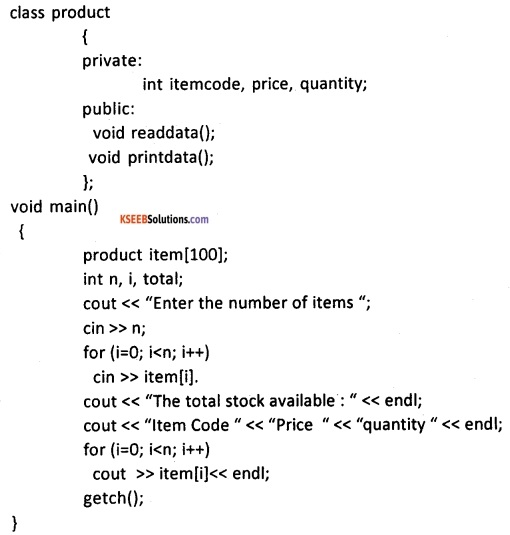Question 9.
A class clock has following members

1. hour
2. minute.

Create member functions
Answer:

1. to initialize the data members
2. display the time
3. to convert hours and minutes to minutes.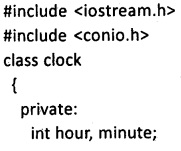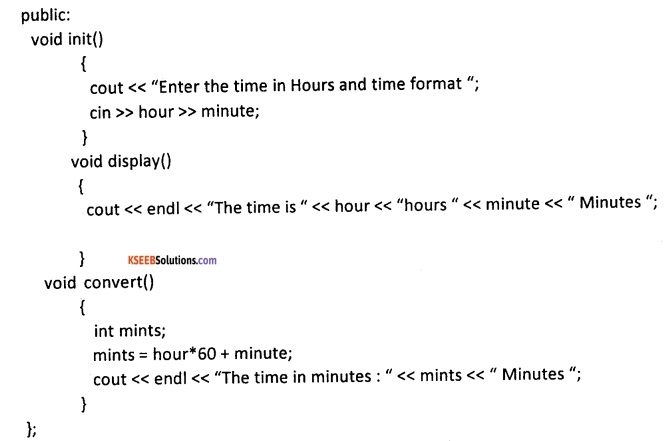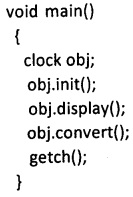Question 10.
Write a program that receives arrival time, departure time and speed of an automobile in kilometers/hour as input to a class. Compute the distance travelled in meters/second and display the result using member functions.
Answer: